The graph shows the relationship between the number of hours that Michelle has been driving and the distance that she has left to travel to

Question

The graph shows the relationship between the number of hours that Michelle has been driving and the distance that she has left to travel to get to her destination.

A graph on a coordinate plane titled Distance Remaining Over Time. The x-axis is labeled time (in hours), numbered 1 to 8, and the y-axis is labeled miles to destination, numbered 50 to 400. A straight line with a negative slope starts at point (0, 350) and ends at point (7, 0).

Which statement is true?

It took Michelle 6 hours to complete the trip.
For each hour that Michelle drove, she traveled an additional 50 miles.
In the first 6 hours, Michelle had traveled a total of 50 miles.
In the first 3 hours, Michelle had traveled a total of 200 mile\

in progress 0
4 months 2021-09-05T06:45:17+00:00 1 Answers 59 views 0

(b) For each hour that Michelle drove, she traveled an additional 50 miles.

Step-by-step explanation:

Given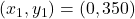—- start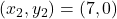— end

Required

Which is true

(a): Journey = 6 hours

This is false, because: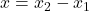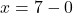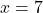—- 7 hours

(b): The average rate is 50 miles per hour

To do this, we calculate the slope (m) using: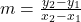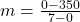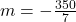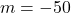This means that the rate is 50 miles driven in 1 hour.

(b) is correct

Others are incorrect